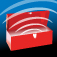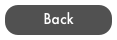RF Toolbox User GuideRF Tools

Fresnel Zone

1. The pattern of electromagnetic radiation that is created by a transmitting station from its antenna to receiving antennas, commonly described as an elongated football shape. The size of zone is dependent on frequency and distance. Obstructions within the fresnel zone will impede the radio transmission. Typically, a minimum acceptable link will have at least 60% of the primary fresnel zone clear of obstructions.

Downtilt Coverage

1. Calculates the inner and outer coverage of an antenna where signal strength is -3dB (half power). Enter antenna height, beamwidth of the antenna, and mechanical or electronic downtilt. Shows distance for inner and outer radius or HORIZON if -3dB signal goes beyond the horizon.

Antenna Downtilt

1. Calculates the downtilt (or Uptilt) required between two antennas in order to align them in a Point to Point Radio link.

2. Enter the heights of the antennas and distance to calculate Angle. Select Angle Button to calculate distance based on antenna heights and a given angle.

dBm / Watt Converter

1. Enter dBm or MilliWatts or Watts and select Done. Calculates other values based on entry. Valid entries:

2. dBm: -80 to +80

3. MilliWatts: 1e-8 to 100m

4. Watts: 1e-11 to 100k

5. Scientific or numeric entries can be used.

Free Space Loss

1. The loss in signal strength of an electromagnetic wave that would result from a line-of-sight path through free space, with no obstacles nearby to cause reflection or diffraction. Enter distance and frequency to calculate Path Loss.

Earth Curvature

1. As the distance of an RF link increases, so does the amount of earth curvature which needs to be calculated into a path analysis. In addition, RF signals are 'bent' slightly as they propagate.  This process is called atmospheric refraction and is referred to as k-factor. A k-factor of 1.33 or 4/3 is normally used to calculate radio waves. A k-factor of 1.0 would be used to calculate earth curvature without any refraction.

Cable Loss

1. The inherent loss of coaxial RF cables due to conductor resistances, absorptive losses in the insulating material and signal leakage between the braids of the outer shield. Enter Frequency, Length of Cable, and select Cable Type from the Cable Picker. Calculates total loss in dB.

2. Calculator uses a fairly accurate but simplified calculation. Check manufacturer specs for exact calculation.

1. Calculates the Fade Margin or Distance or Transmit Power using all aspects of an RF Link. Select type of calculation and then enter remaining values. All values can be entered in positive values. The calculator with convert Cable Loss and RX Sensitivity to negative values.

EIRP

1. Calculates the EIRP (Effective Isotropic Radiated Power) emitted at the Transmit antenna using

3.     plus Antenna Gain

4.     minus Cable Loss.

5. Allows dBm or Watts entry and calculation

Wave Length

1. Calculates either the Wave Length based on a Frequency or Frequency based on a given Wave Length. Enter either value and select Done to Calculate.